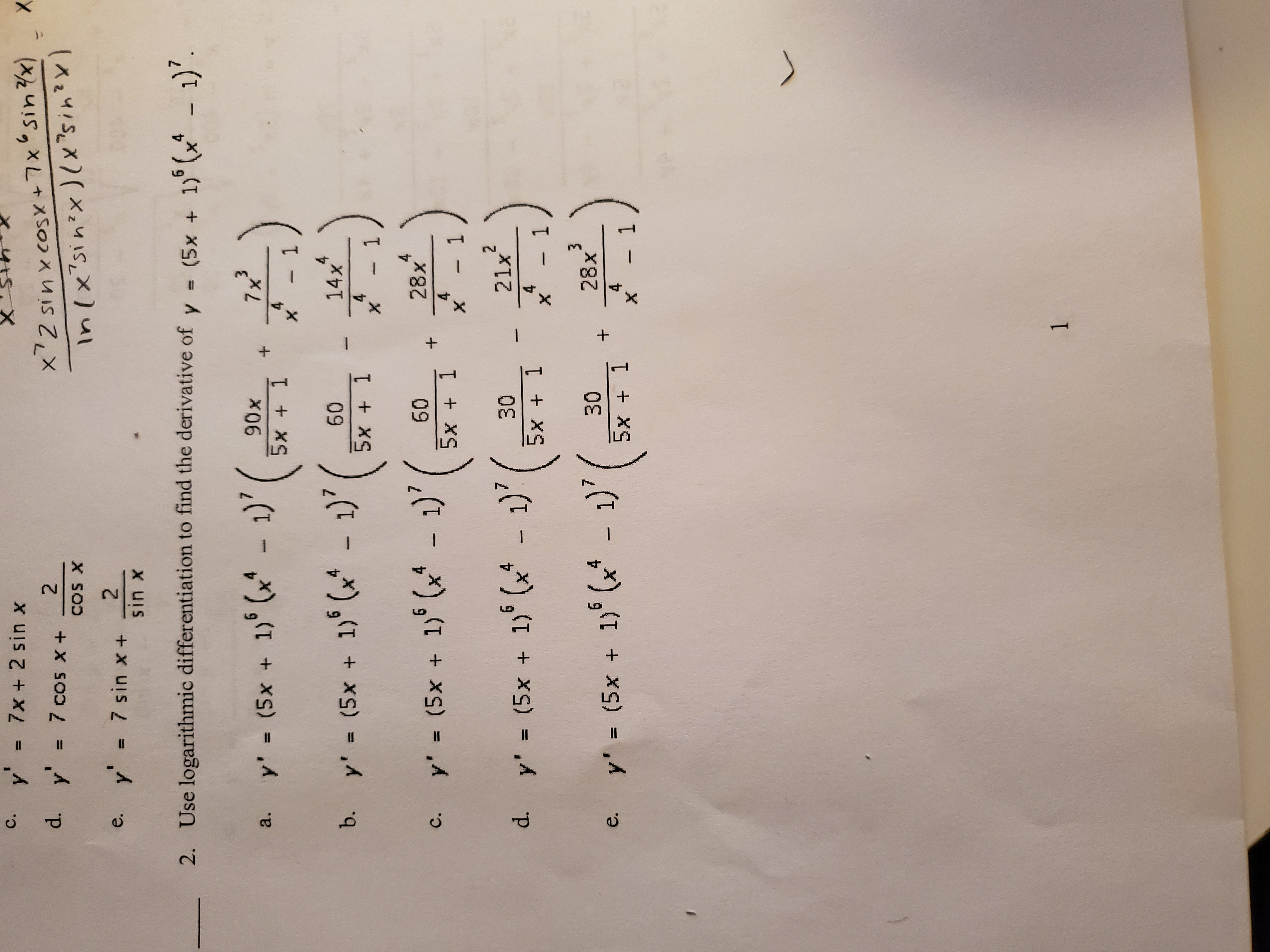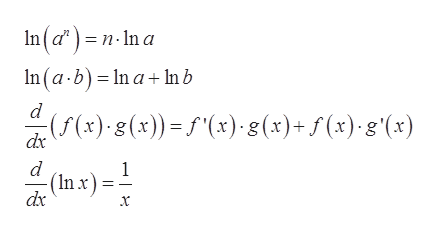y = 7x + 2 sin xс.YXx72 sinxcosx +7 x '5in x)2y'= 7 cos x+d.COS X27 sin x+sin xy'e.4Use logarithmic differentiation to find the derivative of2.- (5x +37x90xy' (5x + 1) (x - 1)a.5x + 14х14x60y' = (5x + 1) (- 1)b.5x 14X1428x60y' (5x + 1) (x - 1)'с.415x- 1X(21x304d. y' (5x +45x 1- 1X328x- 1)5x 1304- (5x + 1) (y' =е.4- 1X(1

Question

Use logarithmic differentiationhelp_outlineImage Transcriptionclosey = 7x + 2 sin x с. YX x72 sinxcosx +7 x '5in x) 2 y'= 7 cos x+ d. COS X 2 7 sin x+ sin x y' e. 4 Use logarithmic differentiation to find the derivative of 2. - (5x + 3 7x 90x y' (5x + 1) (x - 1) a. 5x + 1 4 х 14x 60 y' = (5x + 1) (- 1) b. 5x 1 4 X 1 4 28x 60 y' (5x + 1) (x - 1)' с. 4 1 5x - 1 X ( 21x 30 4 d. y' (5x + 4 5x 1 - 1 X 3 28x - 1) 5x 1 30 4 - (5x + 1) ( y' = е. 4 - 1 X ( 1 fullscreen
Step 1

To determine the logarithmic derivative of the function.

Step 2

Given:

Step 3

Formula used:

...help_outlineImage TranscriptioncloseIn(d)=n-In a n(a-b) In a+In b (f(x) g(x))=f"(x) 8(x)+f(x).g'(x) dx d (Inx) dx 1 fullscreen

Want to see the full answer?

See Solution

Want to see this answer and more?

Our solutions are written by experts, many with advanced degrees, and available 24/7

See Solution
Tagged in

Derivative